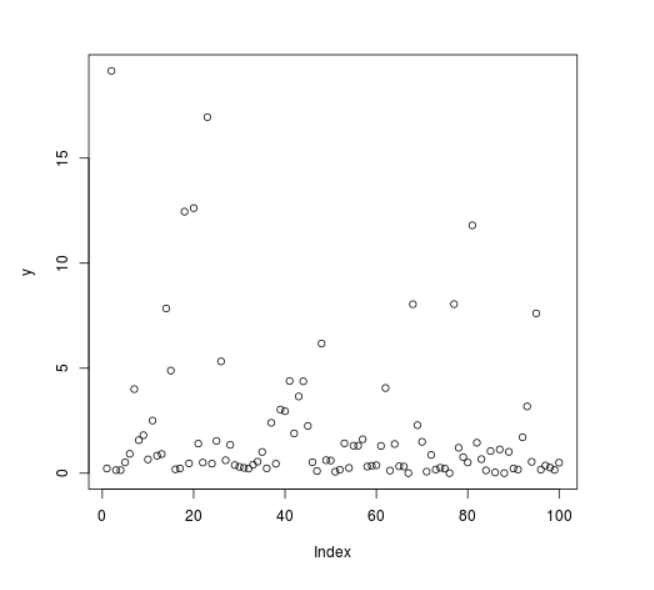# Compute Randomly Drawn F Density in R Programming – rf() Function

`rf()` function in R Language is used to compute random density for F Distribution.

Syntax: rf(N, df1, df2)

Parameters:
N: Sample Size
df: Degree of Freedom

Example 1:

 `# R Program to compute random values ` `# of F Density ` ` `  `# Setting seed for ` `# random number generation ` `set``.seed(``1000``) ` ` `  `# Set sample size ` `N <``-` `20` ` `  `# Calling rf() Function ` `y <``-` `rf(N, df1 ``=` `2``, df2 ``=` `3``) ` `y `

Output:

```   0.2254501 19.1565944  0.1462503  0.1443822  0.5184883  0.9231497
  4.0047848  1.5749135  1.8080354  0.6584719  2.5080273  0.8327536
  0.9125265  7.8437920  4.8880169  0.1858029  0.2296163 12.4537679
  0.4680668 12.6245564
```

Example 2:

 `# R Program to compute random values ` `# of F Density ` ` `  `# Setting seed for ` `# random number generation ` `set``.seed(``1000``) ` ` `  `# Set sample size ` `N <``-` `100` ` `  `# Calling rf() Function ` `y <``-` `rf(N, df1 ``=` `2``, df2 ``=` `3``) ` ` `  `# Plot a graph ` `plot(y) `

Output:My Personal Notes arrow_drop_upCheck out this Author's contributed articles.

If you like GeeksforGeeks and would like to contribute, you can also write an article using contribute.geeksforgeeks.org or mail your article to contribute@geeksforgeeks.org. See your article appearing on the GeeksforGeeks main page and help other Geeks.

Please Improve this article if you find anything incorrect by clicking on the "Improve Article" button below.

Article Tags :

Be the First to upvote.

Please write to us at contribute@geeksforgeeks.org to report any issue with the above content.